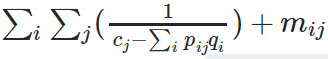# The relationship between NP-hardness and Convexity

Hello, Is there a relationship between NP-hardness and Convexity?
For instance, is it possible for a convex objective function to be an NP-hard problem like a partition problem? If yes, can the results be considered as an approximation of optimal answer?

Copositive Programs are convex and NP-hard. See p.1 of http://sburer.github.io/papers/033-cpchap.pdf .

if you wish further discussion on this, as it is not a CVX-specific matter, I suggest you post elsewhere, such as at https://or.stackexchange.com/ .

As you suggested correctly that my problem is not directly CVX-specific, I asked my question in 2 other forums including https://or.stackexchange.com/. However, no one could answer me and I wish that due to your skill in the convex problems you help me.
My problem is to minimizewhere the q_i is positive always, 0<=p_{ij}<=1, \sum_jp_{ij}=1, the denominator has to be positive and m_{ij} is random positive number, but the problem seems to be a partition problem as the p_{ij} can partition the problem solution into the sets that the ∑_ip_{ij}q_i of the sets is the same. So can the obtained solution be correct?

I’m not even sure what your problem is. Are p_{ij} the only optimization (decision) variables? If so, use inv_pos in CVX.

Yes, the only decision variable is p_{ij}. I know how to model it in CVX but my question is when a problem is inherently NP-Hard and we solve it by cvx, is the solution accurate or not?

I believe that any problem you can model in CVX without use of binary or integer variables is not NP-Hard. Although I am not sure that is the case for problems which don’t satisfy the Slater constraint qualification. Problems which don’t satisfy the Slater constraint qualification invalidate the premise of the primal-dual solvers which CVX calls, and at a minimum, can result in unreliable solution, generally evidenced by a status from the solver that it encountered difficulty or failed.

Fortunately, none of the solvers show the status of failed or inaccurate. My only doubt is that my problem seems to be reducible to a partition problem as there can be two sets of solutions that optimize my problem. Doesn’t it make the solution unreliable in general?

Not as far as i know. If the solution is non-unique, you are at the 'whim" of whichever optimal solution the solver returns.